# SQL Math Functions: ABS, CEIL, FLOOR, ROUND Examples

In the previous tutorials, we covered arithmetic calculations in SQL. Building upon that knowledge, we're now introducing 6 more mathematical functions that enable us to do even more complex calculations!

Let's work through a Bloomberg SQL question that illustrates how these functions are applied in real-world scenarios.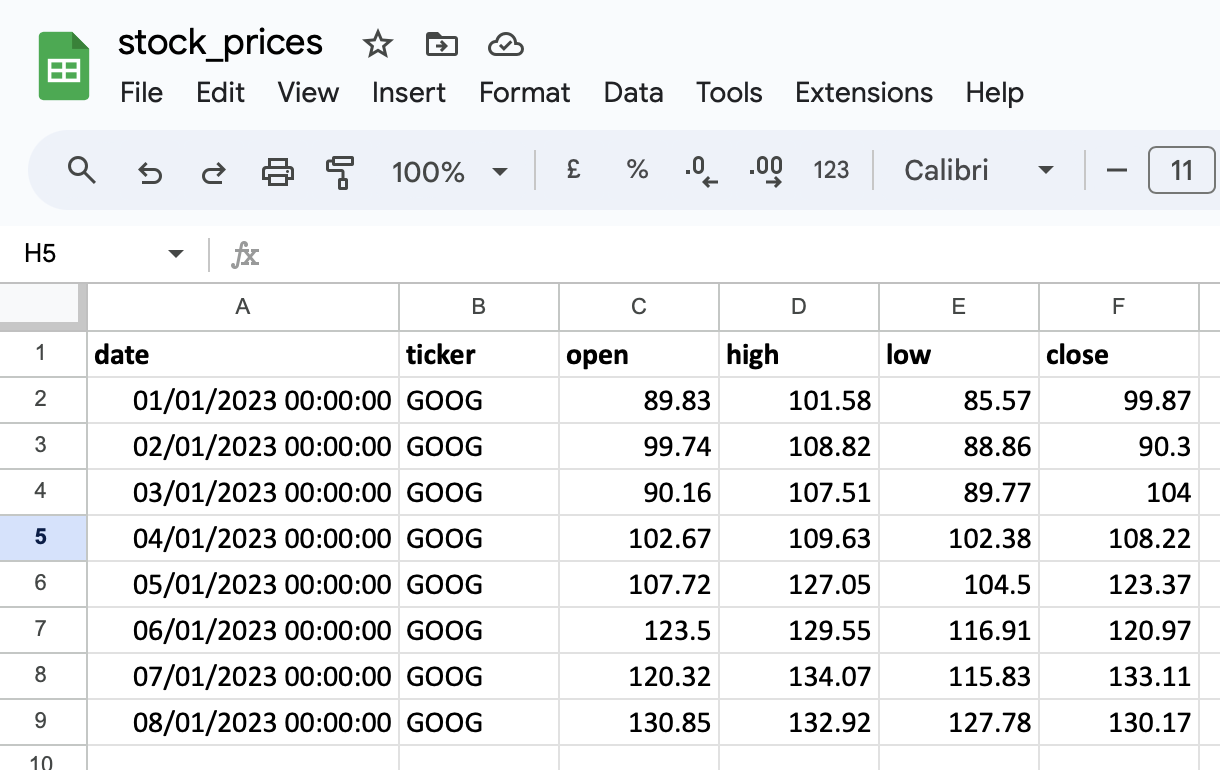### ABS(): Calculating Absolute Differences

Imagine you're tasked with preparing a dashboard showcasing the historical performance of Google's stock (ticker: 'GOOG') throughout 2023. The finance team specifically requests the absolute difference between the opening and closing prices for each trading day.

We can utilize the function which calculates the absolute value of a number, irrespective of its sign.

In this example, we compute the difference between closing and opening prices for each day using the function and returns its absolute value.

See how in the calculation, you end up with a difference of -9.44, including the negative sign? But with the helpful function, you get a positive difference of 9.44 without the negative sign.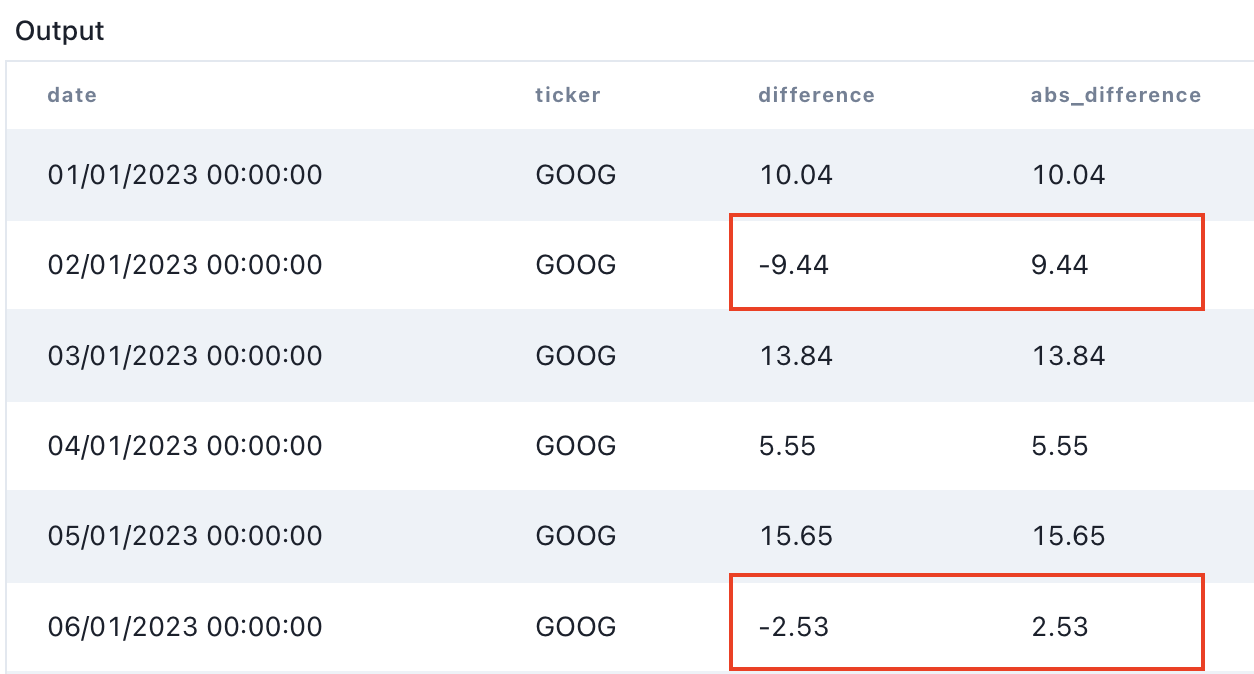### ROUND(): Rounding Numbers

Consider a scenario where you're compiling a report displaying the average closing prices of Apple's stock (ticker: 'AAPL') throughout 2022. To ensure the report's readability, you decide to round the average prices to 2 decimal places.

The function is handy here; it rounds a number to a specified number of decimal places.

In the initial calculation, the output displays with 10 decimal places which honestly just looks unpleasant. However, by using , you specify the number of decimals you prefer, resulting in a neatly rounded up price.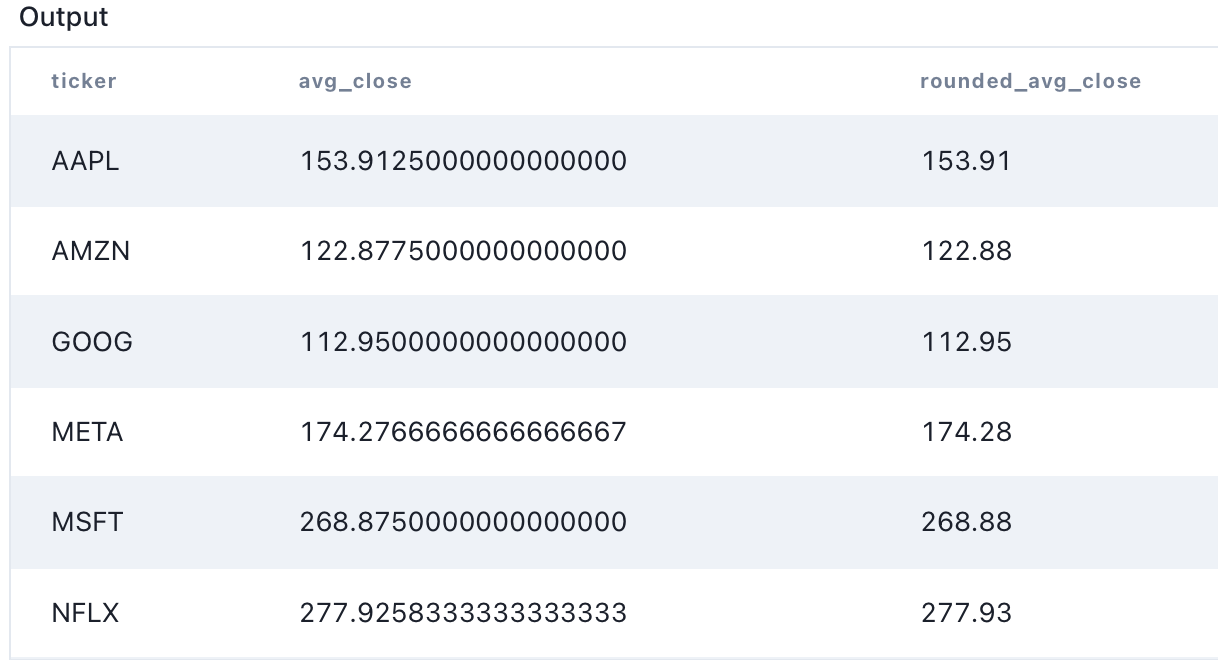We'll cover more about rounding, and the annoyances of integers vs. floating-point decimal numbers in the SQL division tutorial later.

### CEIL() and FLOOR(): Rounding Up and Down

Now, imagine you're analyzing Facebook's stock performance (ticker: 'META') to identify potential trading ranges.

We can utilize the function to round up and the function to round down to the lowest and highest prices.

By employing on the low column, we highlight potential support levels by rounding down the lowest prices. Conversely, with on the high column, we identify potential resistance levels by rounding up the highest prices.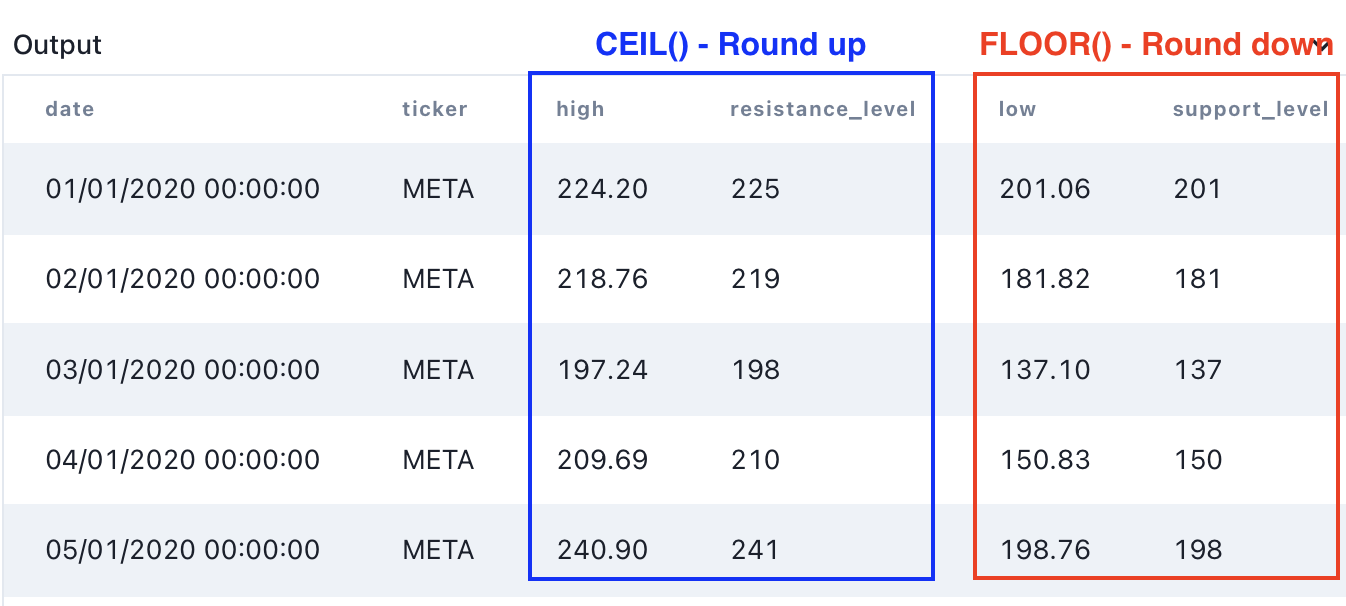## POWER(): Calculating Squared Values

In this scenario, we'll explore the concept of squared values by focusing on the 3 popular tech stocks: Apple ('AAPL'), Google ('GOOG'), and Microsoft ('MSFT'). Although this scenario is simplified for illustrative purposes, it offers a straightforward way to introduce the usage of the function.

The function raises a number to a specified power, allowing us to perform exponential calculations.

In this query, we're leveraging the function to calculate the squared values of the closing prices for the selected tech stocks.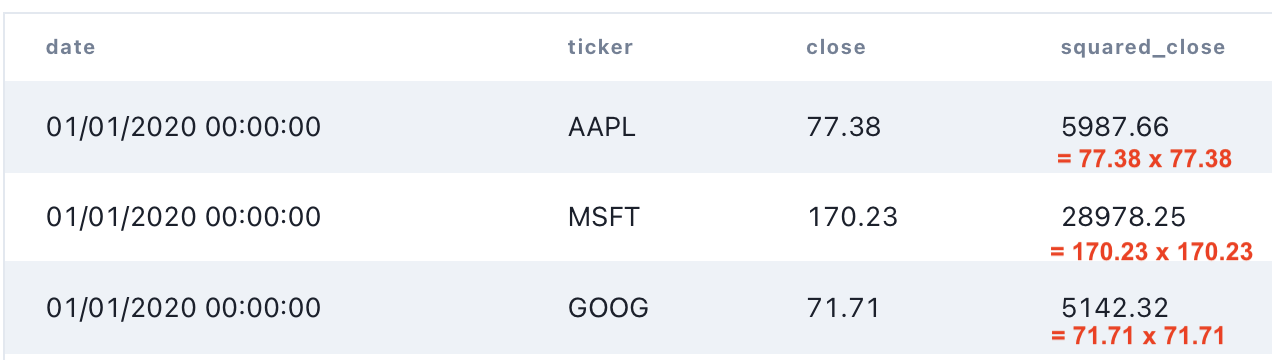For shorthand, in PostgreSQL, we can also just use the operator too!

#### MOD() or %

Imagine you're intrigued by stocks that exhibit a consistent price behavior divisible by 5.

The function or modulo operator calculates the remainder of division between two numbers.

In this example, calculates the remainder when the closing prices are divided by 5. The operator achieves the same result. Both approaches help in identifying stocks with price behaviors divisible by 5.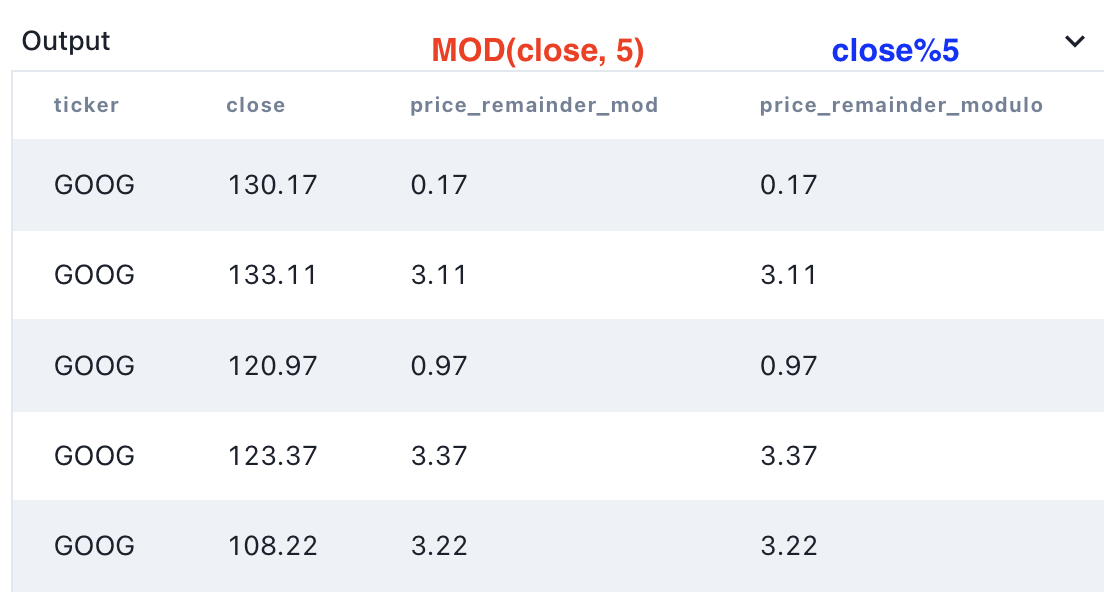### SQL CEIL Practice Exercise

In the following problem, we'll analyze the CVS pharmacy sales dataset, and try to find the per unit cost of medicines made by Merck. We'll round our unit cost up to the nearest dollar, courtesy of .

The output should look like this:

drugunit_cost
FLU KIDS RELIEF11
DHEA14
METHOCARBAMOL18
Keytruda58
Divalproex sodium100

#### What's Next: SQL Division

In the next tutorial, we'll laser focus on SQL division, talk more about tricks to round numbers, and how to between different data-types.

Next Tutorial: SQL Division

Next Lesson

SQL DIVISION ➗